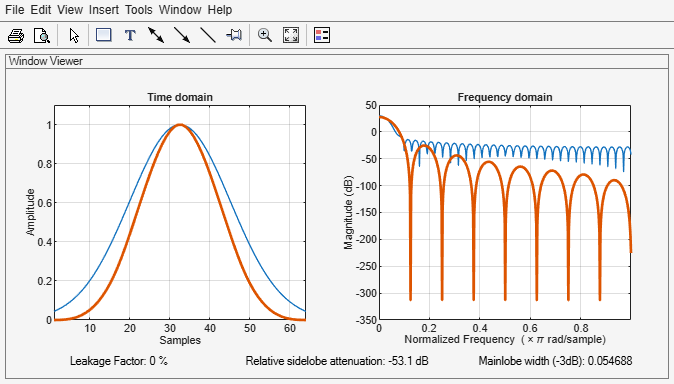# parzenwin

Parzen (de la Vallée Poussin) window

## Syntax

w = parzenwin(L)

## Description

example

w = parzenwin(L) returns the L-point Parzen (de la Vallée Poussin) window.

## Examples

collapse all

Compare 64-point Parzen and Gaussian windows. Display the result using wvtool.

gw = gausswin(64); pw = parzenwin(64); wvtool(gw,pw)## Input Arguments

collapse all

Window length, specified as a real positive scalar.

Data Types: single | double | int8 | int16 | int32 | int64 | uint8 | uint16 | uint32 | uint64

## Output Arguments

collapse all

Parzen window, returned as a column vector of length L. For the equation that defines the Parzen window, see Algorithms.

## Algorithms

Parzen windows are piecewise-cubic approximations of Gaussian windows. Parzen window sidelobes fall off as 1/ω4.

This equation defines the N–point Parzen window over the interval $-\frac{\left(N-1\right)}{2}\le n\le \frac{\left(N-1\right)}{2}$:

$w\left(n\right)=\left\{\begin{array}{cc}1-6{\left(\frac{|n|}{N/2}\right)}^{2}+6{\left(\frac{|n|}{N/2}\right)}^{3}& 0\le |n|\le \left(N-1\right)/4\\ 2{\left(1-\frac{|n|}{N/2}\right)}^{3}& \left(N-1\right)/4<|n|\le \left(N-1\right)/2\end{array}$

 Harris, Fredric J. "On the Use of Windows for Harmonic Analysis with the Discrete Fourier Transform." Proceedings of the IEEE®. Vol. 66, January 1978, pp. 51–83.